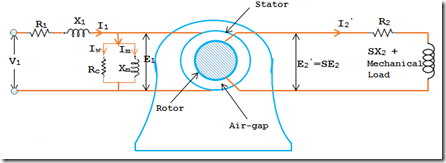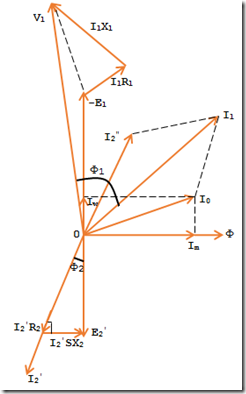# Equivalent Circuit of a Three Phase Induction Motor

In a three phase induction motor the stationary winding is connected to the three phase supply and rotor winding is short circuited. The energy from the stator winding is transferred magnetically to the short circuited rotor winding. Thus the induction motor might be deliberated to be a transformer with secondary winding (rotor short circuited winding). The stator windings refer to the primary of transformer and the rotor windings refer to the secondary of transformer. In view of the comparison of flux and conditions of voltage in a transformer, each can assume that the induction motor equivalent circuit and transformer equivalent circuit will be same. The figure below shows that per phase equivalent circuit on an induction motor.### Stator Circuit:

In stator winding, the actions are very parallel to those in the primary of transformer. The voltage per phase is applied to the stator winding is V1, the stator resistance is R1 and the leakage reactance is X1. The voltage applied to the stator creates a magnetic flux which links the stator as well as the rotor winding i.e. primary and secondary of transformer. As an outcome the self-induced EMF E1 induced in the stator winding and mutually induced EMF E2 is induced in the secondary winding (rotor winding). The drift of stator current I1 causes voltage drops in R1 and X1.
V1 = -E1 + I1 (R1 + jX1)

Once the motor is at no load, the stator windings draw current I0. It has 2 constituents, a) which supplies the no load motor losses and b) magnetizing component Im which set-up magnetic flux in the core and the air gap. The parallel combination of Rc and Xm, therefore, represents the no load motor losses and the production of magnetic flux respectively.
I0 = Iw + Im

### Rotor Circuit:

In the rotor circuit R2 represents the rotor resistance and X2 represents the standstill rotor reactance. At any slip S, the reactance of rotor will be SX2. The induced voltage per phase in the rotor circuit is E2 = SE2 = SKE1. The whole EMF E2 is employed in circulating the rotor current I2, since the rotor winding is short circuited.
E2 = I2 (R2 + jSX2)

The rotor current I2 is reflected as I2 (KI2) in the stator. The sum of Phasor is I2 and I0 gives the stator current I1.
It is much important is to note that the transformer input and output are electrical. Conversely in an induction motor the input to the rotor and stator are electrical but the motor output (i.e. from rotor) is mechanical. To simplify calculations it is required and essential to replace the mechanical load. Then we have the transformer equivalent circuit to the induction motor.
It might be noted that though the stator current and rotor current frequencies are different, however the magnetic fields due to them rotate at synchronous speed Ns. The stator current creates a magnetic flux which rotates at synchronous speed Ns. At slip S, the speed of rotation of the rotor field kin to the rotor surface in the direction of rotation of the rotor is
= 120f/P
= 120SF/P
= SNs

But the rotor is rotating at a speed of N relative to the stator core. Therefore, the speed of rotor field comparative to stator core.
= SNs + N
= (Ns – N) + N = Ns

In consequence no matter what the value of slip, the magnetic fields of the stator and rotor are synchronous with each other. As a result the three phase induction motor can be considered as being equivalent to a transformer having an air gap splitting the iron portions of the magnetic circuit carrying the primary winding and secondary winding. The Figure below shows that phasor diagram of an induction motor.2 CommentsComments

#### Read Comment Policy We have Zero Tolerance to Spam. Chessy Comments and Comments with Links will be deleted immediately upon our review.

1.Great tutorial on induction motor. It would be much helpful to see how torque comes in picture and torque-speed curve derived too.
Great! Thanks a lot.

2.Can you explain why E1 is negative? Based on the equivalent model, it seems that E1 should be positive. Thanks.